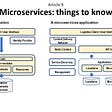# 3 C++ For Loop Implementations

TL;DR —The goal is to quickly review C++ syntax needed to iterate through a vector using a `for` loop. We will use an index, pointer, and iterator. You will find the full code listing at the bottom of this post.

Let’s begin by instantiating an integer vector named `ageVector`.

`vector<int> ageVector = { 10,20,30,40,50 };`

## 1. C++ for loop INDEX implementation.

`for (int i = 0; i < ageVector.size(); i++) {        cout << ageVector[i] << " ";}`

Initialize an integer `i = 0`, this will be used as an index. Then set the loop condition `i < ageVector.size()` where the index is incremented by one, `i++`. In the code block, access the element contents using an access operator, here we use `ageVector[i]`.

## 2. C++ for loop POINTER implementation.

`for (int* ptr = &ageVector;      ptr <= &ageVector[ageVector.size()-1];      ptr++) {        cout << *ptr << " ";    }`

First we declare an integer pointer and set it to the address of the first element in the vector, `ptr = &ageVector`. Then set the loop condition to compare the pointer against the address of the last element, `ptr <= &ageVector[ageVector.size()-1]`. Finally, use pointer arithmetic to increment to the next address, `ptr++`.

To access the contents, remember to dereference the pointer. In the code block, we do this with `*ptr`.

## 3. C++ for loop ITERATOR implementation.

`for (vector<int>::iterator itr = ageVector.begin();      itr < ageVector.end();      itr++) {        cout << *itr << " ";    }`

Declare an integer vector iterator and set it to the beginning of the vector, `vector<int>::iterator itr = ageVector.begin()`. Then set the loop condition to compare the iterator against the past-the-end element, `itr < ageVector.end()`. Finally, use `itr++` to increment to the next vector element.

To access the contents, remember to dereference the iterator. In the code block, we do this with `*itr`.

That’s our quick review. Here is the full code listing.

`#include <iostream>#include <vector>using namespace std;int main(void){vector<int> ageVector = { 10,20,30,40,50 };cout << "\n ***for loop with index" << endl;for (int i = 0; i < ageVector.size(); i++) {        cout << ageVector[i] << " ";}cout << "\n\n ***for loop with pointer" << endl;for (int* ptr = &ageVector; ptr <= &ageVector[ageVector.size()-1]; ptr++) {        cout << *ptr << " ";}    cout << "\n\n ***for loop with iterator" << endl;for (vector<int>::iterator itr = ageVector.begin(); itr < ageVector.end(); itr++) {        cout << *itr << " ";}cout << "\n\n ***Enjoy" << endl;return 0;}`

--

--

--

## More from John Redden

Professor and Author

Love podcasts or audiobooks? Learn on the go with our new app.

## Worksession Recap: 01/08## Social factors drive your technology muscle and software architecture. Don't underestimate them.## Point & Click to Move in Unity 2021## Secure JWT authentication against both XSS and XSRF (Vue.js + Django REST)## Kotlin Interface## Must we convert monoliths to microservices?## 402. Remove K Digits## John Redden

Professor and Author

## Serial Port programming Tutorial using Java SE for Embedded Developers## JS Functional Programming Simplified## Setup up environment for C Programming